# Video Calc I Tutor

価格 ｼﾞｬﾝﾙ サイズ このアプリは現在ストアで閲覧することができません。 240円 ダウンロード教育 -- iPREPpress LLC ｼﾞｬﾝﾙ別：---総合：--- -- 評価が取得できませんでした。 iOS 以降が必要です。 iPad 対応。 2019-08-26　このアプリは現在ストアから情報が取得できません。削除された可能性があります。
Video Calc I Tutor by Larry Green

With 24 MAJOR CATEGORIES & 87 VIDEO LECTURES totaling over 7 HOURS of lessons, Video Calc I Tutor covers first part of a four part series in college calculus curriculum in video, tutorial form.

* These 87 VIDEOS are in app, not streamed from Internet. You can use this product anywhere.

Video Calc I Tutor lessons include:
Limits:
* A Preview of Calculus
* Limits Graphically & Numerically
* Limits Analytically
* Continuity & one Sided Limits
* Asymptotes

Derivatives
* Limit Definition of Derivative
* Basic Derivative Rules
* Product & Quotient Rules
* Chain Rule
* Implicit Differentiation

Applications of Derivative
* Related Rates
* Critical Points & Extrema on an Interval
* Rolle's orem & Mean Value orem
* Increasing & Decreasing Functions & First Derivative Test
* Concavity & Second Derivative Test
* Limits at Infinity
* Calculus & Curve Sketching
* optimization
* Newton's Method
* Differentials
o Differentials & Comparing Dy & dy
o Using Differentials to Approximate a Square Root
o Using Differentials Graphically to Approximate a Function Value
o Using Differentials to Approximate Percent Propagated Error
o Using Differentials to Prove Relative Errors are Equal in Magnitude

Antiderivatives & Indefinite Integration
* Antiderivatives
* Area
* Definite Integral
o Looking at a Graph & Setting Up a Definite Integral
o Using Geometry to Evaluate a Definite Integral
o Using Properties of Definite Integral
o Integrating a Piecewise Linear Function Using Its Graph
* Fundamental Thorem of Calculus
o Using 1st Fundamental orem of Calculus
o Finding Area of a Region
o Finding Average Value
o Using 2nd Fundamental orem of Calculus
o Chain Rule & 2nd Fundamental orem
o Integration Props & 2nd FTC
o Sketching Graph of y = f (x) Given Graph of y = f '(x)
* Substitution
* Numerical Integration
o Using Trapezoidal Rule to Approximate an Integral
o Using Simpson's Rule to Approximate an Integral
o Finding Maximum Error in Simpson's Rule
o Find n to Approximate an Integral with Trapezoidal Rule

***
Each audio and video study guide app has been meticulously created by iPREPpress LLC, a leader in educational iPod, iPhone and mobile device development and distribution.

Now Loading...
オススメ！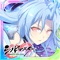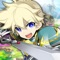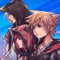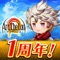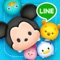お得情報ピックアップ！80%値引中84%値引中34%値引中40%値引中50%値引中「iPhone & iPad アプリランキング」は、最新かつ詳細なアプリ情報をご紹介しているサイトです。 お探しのアプリに出会えるように様々な切り口でページをご用意しております。
メニュー」よりぜひアプリ探しにお役立て下さい。

Presents by \$\$308413110 　　　スマホからのアクセスにはQRコードをご活用ください。 →

Now loading...